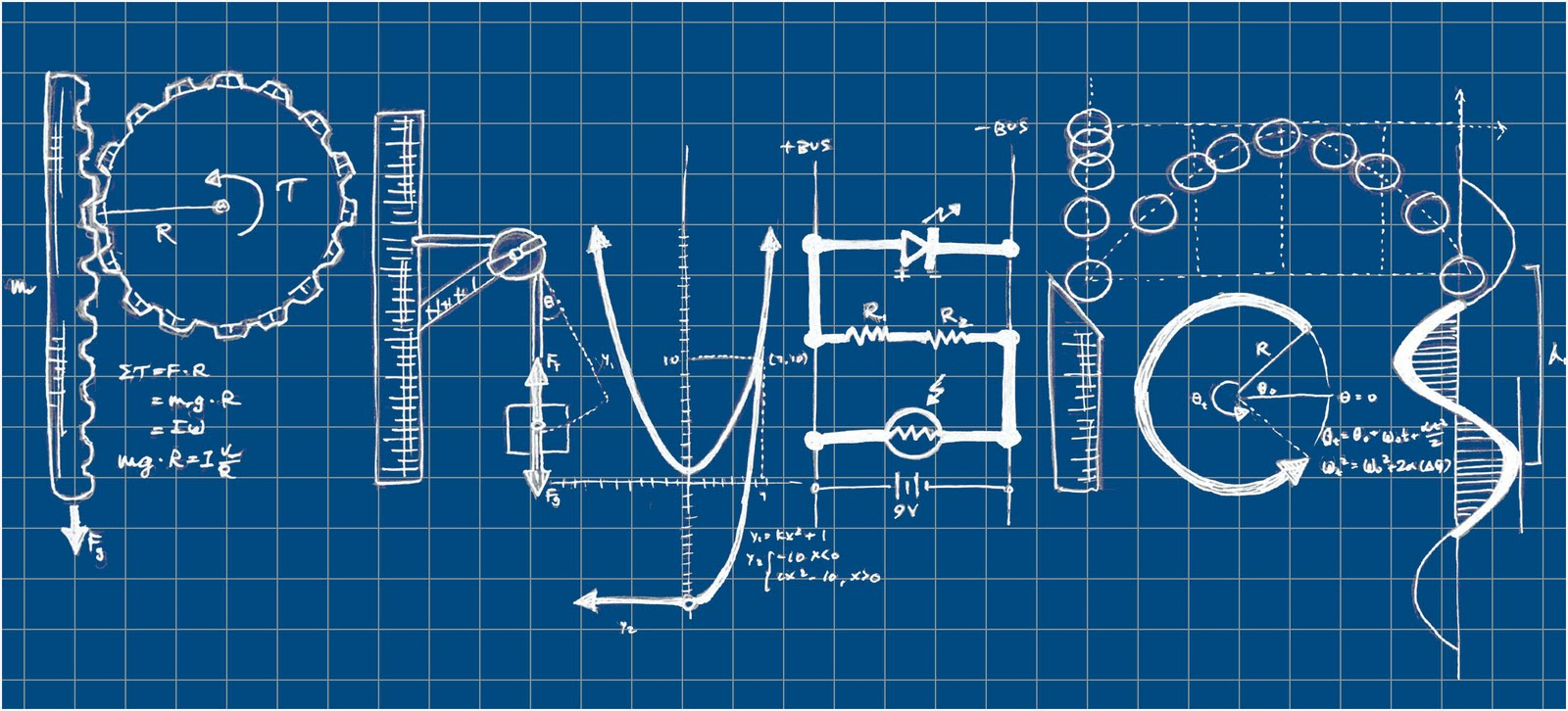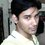# Debates on Electrostatics #1 (All questions from H.C.V)1. The charge on a proton is $\displaystyle +1.6\times { 10 }^{ -19 }C$ and that on an electron is $\displaystyle -1.6\times { 10 }^{ -19 }C$. Does it mean than the electron has a charge $\displaystyle 3.2\times { 10 }^{ -19 }C$ less that the charge of a proton?

2. Consider two particles A and B having equal charges and placed at some distance. The particle A is slightly displaced towards B. Does the force on B increase as soon as the particle A is displaced ? Does the force on the particle A increase as soon as it is displaced?

3. Does the force on a charge due to another charge depend on the charges present nearby?Note by Soumo Mukherjee
5 years, 10 months ago

This discussion board is a place to discuss our Daily Challenges and the math and science related to those challenges. Explanations are more than just a solution — they should explain the steps and thinking strategies that you used to obtain the solution. Comments should further the discussion of math and science.

When posting on Brilliant:

• Use the emojis to react to an explanation, whether you're congratulating a job well done , or just really confused .
• Ask specific questions about the challenge or the steps in somebody's explanation. Well-posed questions can add a lot to the discussion, but posting "I don't understand!" doesn't help anyone.
• Try to contribute something new to the discussion, whether it is an extension, generalization or other idea related to the challenge.

MarkdownAppears as
*italics* or _italics_ italics
**bold** or __bold__ bold
- bulleted- list
• bulleted
• list
1. numbered2. list
1. numbered
2. list
Note: you must add a full line of space before and after lists for them to show up correctly
paragraph 1paragraph 2

paragraph 1

paragraph 2

[example link](https://brilliant.org)example link
> This is a quote
This is a quote
    # I indented these lines
# 4 spaces, and now they show
# up as a code block.

print "hello world"
# I indented these lines
# 4 spaces, and now they show
# up as a code block.

print "hello world"
MathAppears as
Remember to wrap math in $$ ... $$ or $ ... $ to ensure proper formatting.
2 \times 3 $2 \times 3$
2^{34} $2^{34}$
a_{i-1} $a_{i-1}$
\frac{2}{3} $\frac{2}{3}$
\sqrt{2} $\sqrt{2}$
\sum_{i=1}^3 $\sum_{i=1}^3$
\sin \theta $\sin \theta$
\boxed{123} $\boxed{123}$

Sort by:

1. The charges are the same but of opposite polarity. Both will apply force of same magnitude on a unit positive charge but one is attractive an other repulsive.
2. As the electromagnetic waves travel with speed of light, so it will take a little time for force to change.
3. Not at all

- 5 years, 6 months ago